# Quadruped Robot Locomotion using Deep Reinforcement Learning

This project is the final project of ESE 650 Learning in Robotics at the University of Pennsylvania.In this project, I used Deep Reinforcement Learning (DRL) to train an agent to control the locomo...

This project is the final project of ESE 650 Learning in Robotics at the University of Pennsylvania.

In this project, I used Deep Reinforcement Learning (DRL) to train an agent to control the locomotion of a quadruped robot. The DRL algorithm used in this project is Proximal Policy Optimization (PPO). The simulation environment was developed using Unity, and ML-Agents was used for training. The reward function consists of:

• Inclination Reward, where $h_0$ is the robot height, and $h$ is the current height of the robot:

$$R_i = s_i (h_0 - 0.5h) ^ {p_i}$$

• Height Reward, where $h$ is the current robot height, and $\overline{h}$ is the average robot height:

$$R_h = s_h (1-\left|\overline{h}-h\right|)^{p_h}$$

• Walking Direction Reward, where $\theta_w$ is the walking direction of the robot:

$$R_w = s_w (1-(\theta_w-\theta_{target}))^{p_w}$$

• Looking Direction Reward, where $\theta_w$ is the looking direction of the robot, we want to robot to look ahead:

$$R_l = s_l (1-\theta_l)^{p_l}$$

• Speed Punishment, where $v$ is the velocity, return the normalized velocity:

$$R_v = -s_v ||v-v_{target}||$$

To mitigate the problem of reward sparsity and speed up training, I recorded a demonstration using a simple harmonic oscillator and designed a three-stage curriculum to guide the training. Below are some GIFs of the demonstration:

Forward Backward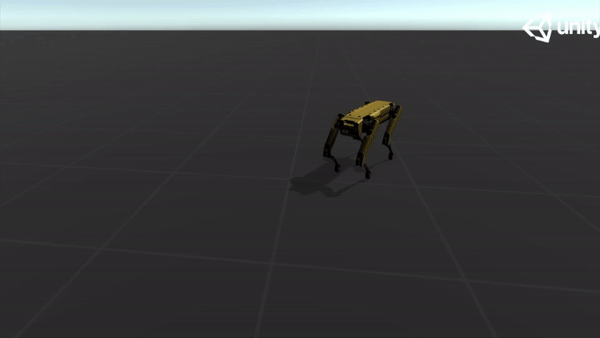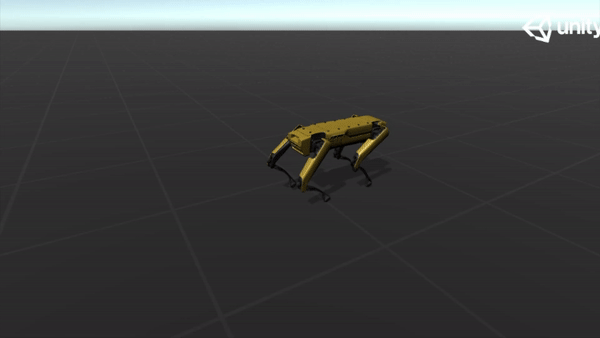Left Right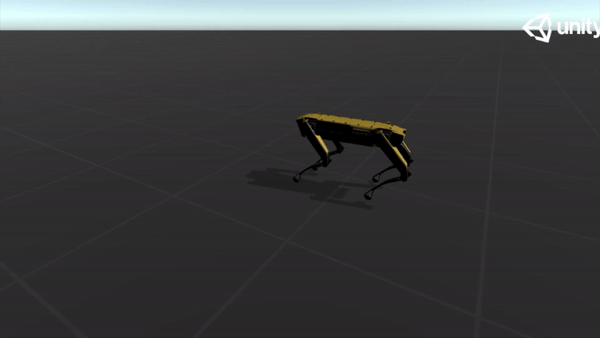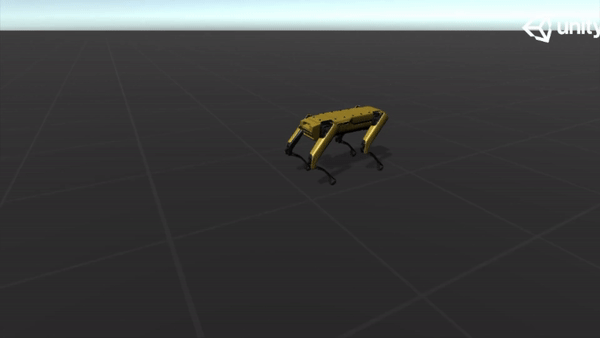The first stage in the curriculum is imitation, which is using behavior cloning to mimic the demonstration. The agent is controlled by fixed speed, walk and look directions. Behavior cloning is applied using the demonstration generated from the oscillator with 0.5 strength. The strength of the extrinsic reward signal is set to 0.1. The first stage is executed for 10 million steps to learn from the demonstration while avoiding overfitting. The purpose of the second stage is to adapt to dynamic speed. In the second stage, the strength of the extrinsic reward is set to 1. The agent is trained for 25 million steps in this stage. The purpose of the third stage is to adapt to different terrain structures and dynamic directions. In the third stage, the terrain is more challenging and unstable. Moreover, the input speed, walk and look direction are random variables that refresh every 10 seconds. The agent is trained for 30 million steps in this stage.

Below are some GIFs of learned policy from various training stages:

Steps Imitation Steps Dynamic Speed Steps Challenging Terrain
5M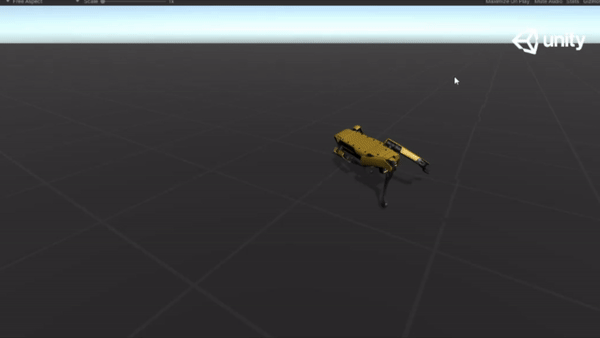20M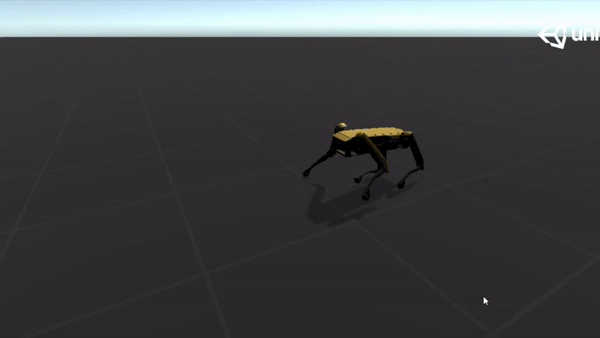35M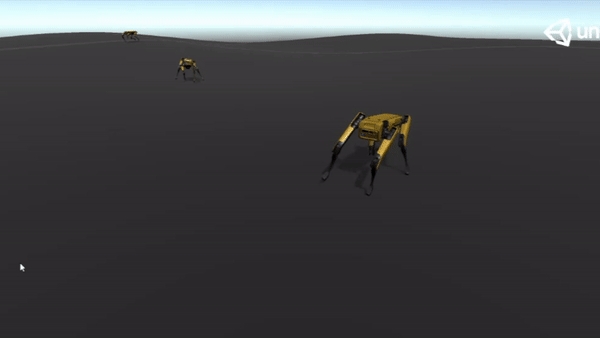10M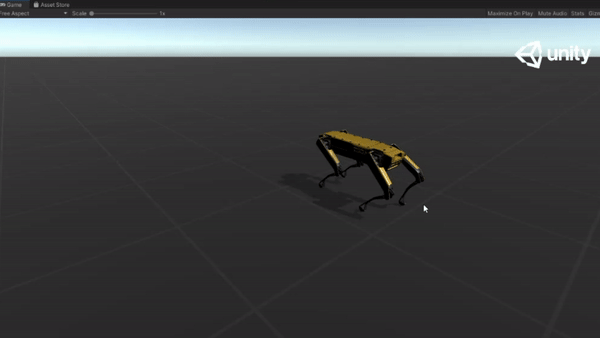30M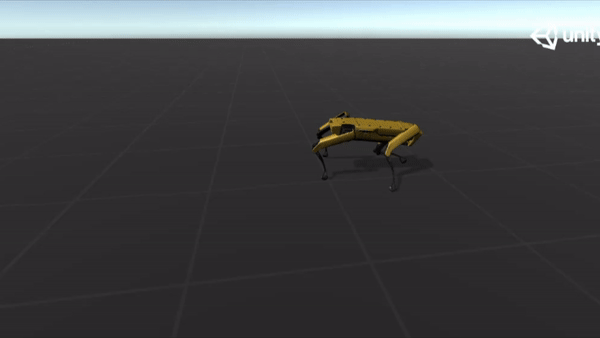65M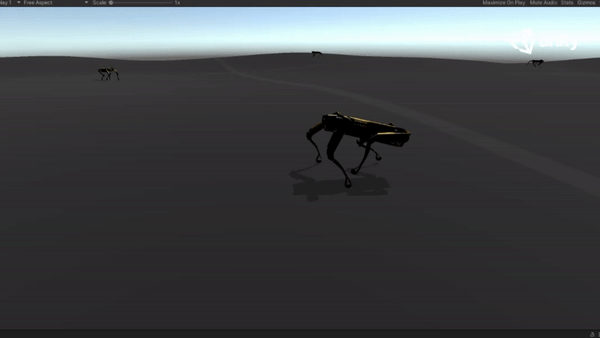After training, the learned policy was tested on four different unseen terrains to evaluate the performance. More detailed information can be found in the report.